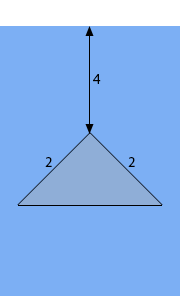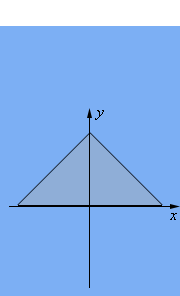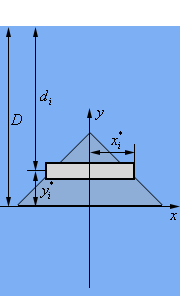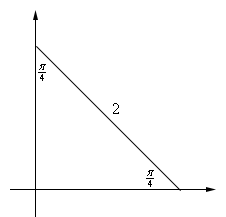Paul's Online Notes
Home / Calculus II / Applications of Integrals / Hydrostatic Pressure and Force
Show Mobile Notice Show All Notes Hide All Notes
Mobile Notice
You appear to be on a device with a "narrow" screen width (i.e. you are probably on a mobile phone). Due to the nature of the mathematics on this site it is best views in landscape mode. If your device is not in landscape mode many of the equations will run off the side of your device (should be able to scroll to see them) and some of the menu items will be cut off due to the narrow screen width.

### Section 8.4 : Hydrostatic Pressure and Force

3. Find the hydrostatic force on the plate submerged in water as shown in the image below. The plate in this case is the top half of a diamond formed from a square whose sides have a length of 2.

Consider the top of the blue “box” to be the surface of the water in which the plate is submerged. Note as well that the dimensions in the image will not be perfectly to scale in order to better fit the plate in the image. The lengths given in the image are in meters.Show All Steps Hide All Steps

Hint : Start off by defining an “axis system” for the figure.
Start Solution

The first thing we should do is define an axis system for the portion of the plate that is below the water.In this case since we had the top half a diamond formed from a square it seemed convenient to center the axis system in the middle of the diamond.

It is always useful to define some kind of axis system for the plate to help with the rest of the problem. There are lots of ways to actually define the axis system and how we define them will in turn affect how we work the rest of the problem. There is nothing special about one definition over another but there is often an “easier” axis definition and by “easier’ we mean is liable to make some portions of the rest of the work go a little easier.

Hint : At this point it would probably be useful to break up the plate into horizontal strips and get a sketch of a representative strip.
Show Step 2

As we did in the notes we’ll break up the plate into $$n$$ horizontal strips of width $$\Delta \,y$$ and we’ll let each strip be defined by the interval $$\left[ {{y_{i - 1}},\,\,{y_i}} \right]$$ with $$i = 1,2,3, \ldots n$$. Finally, we’ll let $$y_i^*$$ be any point that is in the interval and hence will be some point on the strip.

Below is yet another sketch of the plate only this time we’ve got a representative strip sketched on the plate. Note that the strip is “thicker” than the strip really should be but it will make it easier to see what the strip looks like and get all of the appropriate lengths clearly listed.We’ve got a few quantities to determine at this point. To do that it would be convenient to have an equation for one of the sides of the plate. Let’s take a look at the side in the first quadrant. Here is a quick sketch of that portion of the plate.Now, as noted above we have the top half of a diamond formed from a square and so we can see that the “triangle” formed in the first quadrant by the plate must be an isosceles right triangle whose hypotenuse is 2 and the two interior angles other than the right angle must be $$\frac{\pi }{4}$$. Therefore, the bottom/left side of the triangle must be,

${\mbox{side}} = 2\cos \left( {\frac{\pi }{4}} \right) = \sqrt 2$

This means we know that the $$x$$ and $$y$$-intercepts are $$\left( {\sqrt 2 ,0} \right)$$ and $$\left( {0,\sqrt 2 } \right)$$ respectively and so the equation for the line representing the hypotenuse must be,

$y = \sqrt 2 - x$

Okay, let’s get the various quantities in the figure.

We’ll start with $$x_i^*$$ . This we can get directly from the equation above by acknowledging that if we are at $$x_i^*$$ then the $$y$$ value must be $$y_i^*$$. In other words, plugging these into the equation and solving gives,

$y_i^* = \sqrt 2 - x_i^*\hspace{0.25in}\hspace{0.25in} \to \hspace{0.25in}\hspace{0.25in}x_i^* = \sqrt 2 - y_i^*$

Notice as well that the width of each strip in terms of $$y_i^*$$ is then,

$2x_i^* = 2\left( {\sqrt 2 - y_i^*} \right)$

Next, let’s get $$D$$. First we can see that $$D$$ is the distance from the surface of the water to the $$x$$-axis in our figure. We know that the distance from the surface of the water to the top of the plate is 4 meters. Also, we found above that the top point of the plate is a distance of $$\sqrt 2$$ above the $$x$$-axis. So, we then have,

$D = 4 + \sqrt 2$

Finally, the depth of each strip below the surface of the water is,

${d_i} = D - y_i^* = 4 + \sqrt 2 - y_i^*$
Hint : What is the hydrostatic pressure and force on the representative strip?
Show Step 3

We’ll assume that the strip is sufficiently thin so the hydrostatic pressure on the strip will be constant and is given by,

${P_i} = \rho g{d_i} = \left( {1000} \right)\left( {9.81} \right)\left( {4 + \sqrt 2 - y_i^*} \right) = 9810\left( {4 + \sqrt 2 - y_i^*} \right)$

This, in turn, means that the hydrostatic force on each strip is given by,

\begin{align*}{F_i} = {P_i}{A_i} & = \left[ {9810\left( {4 + \sqrt 2 - y_i^*} \right)} \right]\left[ {\left( {2x_i^*} \right)\left( {\Delta \,y} \right)} \right]\\ & = \left[ {9810\left( {4 + \sqrt 2 - y_i^*} \right)} \right]\left[ {2\left( {\sqrt 2 - y_i^*} \right)\left( {\Delta \,y} \right)} \right]\\ & = 19620\left( {4 + \sqrt 2 - y_i^*} \right)\left( {\sqrt 2 - y_i^*} \right)\left( {\Delta \,y} \right)\end{align*}
Hint : How can we use the result from the previous step to approximate the total hydrostatic force on the plate and how can we modify that to get an expression for the actual hydrostatic force on the plate?
Show Step 4

We can now approximate the total hydrostatic force on plate as the sum off the force on each of the strips. Or,

$F \approx \sum\limits_{i = 1}^n {19620\left( {4 + \sqrt 2 - y_i^*} \right)\left( {\sqrt 2 - y_i^*} \right)\left( {\Delta \,y} \right)}$

Now, we can get an expression for the actual hydrostatic force on the plate simply by letting $$n$$ go to infinity.

Or in other words, we take the limit as follows,

$F = \mathop {\lim }\limits_{n \to \infty } \sum\limits_{i = 1}^n {19620\left( {4 + \sqrt 2 - y_i^*} \right)\left( {\sqrt 2 - y_i^*} \right)\left( {\Delta \,y} \right)}$
Hint : You do recall the definition of the definite integral don’t you?
Show Step 5

Finally, we know from the definition of the definite integral that this is nothing more than the following definite integral that we can easily compute.

\begin{align*}F & = \int_{0}^{{\sqrt 2 }}{{19620\left( {4 + \sqrt 2 - y} \right)\left( {\sqrt 2 - y} \right)dy}}\\ & = \int_{0}^{{\sqrt 2 }}{{19620\left( {{y^2} - \left( {4 + 2\sqrt 2 } \right)y + 2 + 4\sqrt 2 } \right)dy}}\\ & = \left. {19620\left( {\frac{1}{3}{y^3} - \left( {2 + \sqrt 2 } \right){y^2} + \left( {2 + 4\sqrt 2 } \right)y} \right)} \right|_0^{\sqrt 2 } = \require{bbox} \bbox[2pt,border:1px solid black]{{96,977.9N}}\end{align*}